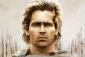# Concavity calculus

4 posts / 0 new
ケネス ケネスConcavity calculus

Here's my question:
Example: examine the function
Y=x(x-1)^3
Y'=3x(x-1)^2+(x-1)^3=(x-1^2(4x-1)
Y"=4(x-1)^2+2(x-1)(4x-1) ... How did they get 4(x-1)^2+2(x-1)(4x-1)?

Tags:
Jhun Vert$y = x(x - 1)^3$

$y' = 3x(x - 1)^2 + (x - 1)^3$

$y' = (x - 1)^2 [ \, 3x + (x - 1) \, ]$

$y' = (x - 1)^2 (4x - 1)$

Use $d(uv) = u \, dv + v \, du$

$u = (x - 1)^2$     →     $du = 2(x - 1)$

$v = 4x - 1$   →     $dv = 4$

$y'' = (x - 1)^2 (4) + (4x - 1)[ \, 2(x - 1) \, ]$

$y'' = 4(x - 1)^2 + 2(4x - 1)(x - 1)$   ←   this is your question

You can also go further by factoring...
$y'' = 2(x - 1) [ \, 2(x - 1) + (4x - 1) \, ]$

$y'' = 2(x - 1)(6x - 3)$

ケネス ケネスLastly, the next equation says that 6(x-1)(2x-1). How did they get 6(x-1)(2x-1)?

AlexanderFrom y'' = 2(x − 1)(6x − 3), factor 3 from (6x - 3) and it will become y'' = 2(x − 1)(3)(2x − 1).

• Mathematics inside the configured delimiters is rendered by MathJax. The default math delimiters are $$...$$ and $...$ for displayed mathematics, and $...$ and $...$ for in-line mathematics.